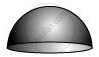# Find the total surface area of a hemisphere of radius 10 cm. - Mathematics

Find the total surface area of a hemisphere of radius 10 cm. [Use π = 3.14]

#### SolutionRadius (r) of hemisphere = 10 cm

Total surface area of hemisphere = CSA of hemisphere + Area of circular end of hemisphere

= 2πr2 + πr2

= 3πr2

= [3 x 3.14 x (10)2] cm2

= 942 cm2

Therefore, the total surface area of such a hemisphere is 942 cm2.

Concept: Surface Area of a Sphere
Is there an error in this question or solution?

#### APPEARS IN

NCERT Class 9 Maths
Chapter 13 Surface Area and Volumes
Exercise 13.4 | Q 3 | Page 225